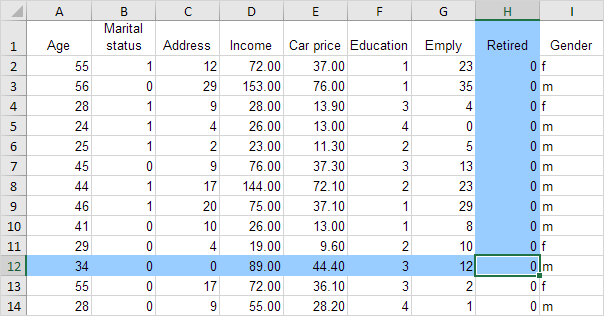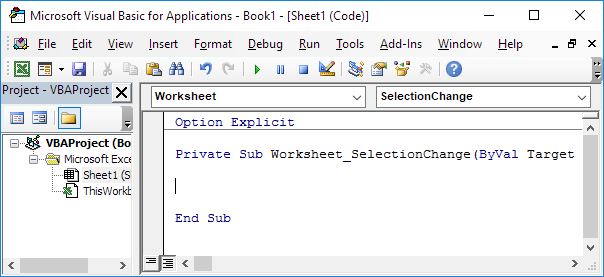# Highlight Active Cell

Below we will look at a program in Excel VBA that highlights the row and column of the Active Cell (selected cell). This program will amaze and impress your boss.

Situation:Each time we change the Active Cell on Sheet1, a macro needs to be executed. You can achieve this by creating a Worksheet SelectionChange Event.

1. Open the Visual Basic Editor.

2. Double click on Sheet1 (Sheet1) in the Project Explorer.

3. Choose Worksheet from the left drop-down list. Choose SelectionChange from the right drop-down list.Add the following code lines to the Worksheet SelectionChange Event:

4. We declare four variables of type Integer. One named rowNumberValue, one named columnNumberValue, one named i and one named j.

Dim rowNumberValue As Integer, columnNumberValue As Integer, i As Integer, j As Integer

5. First, we add the line which changes the background color of all cells to 'No Fill'.

Cells.Interior.ColorIndex = 0

6. We initialize the variable rowNumberValue with the row number of the Active Cell and the variable columnNumberValue with the column number of the Active Cell.

rowNumberValue = ActiveCell.row
columnNumberValue = ActiveCell.column

7. We highlight the column blue. That is: all the cells with row number smaller or equal to rowNumberValue and column number equal to columnNumberValue

For i = 1 To rowNumberValue
Cells(i, columnNumberValue).Interior.ColorIndex = 37
Next i

Explanation: for this example (see picture above), rowNumberValue equals 12 and columnNumberValue equals 8. The code lines between For and Next will be executed twelve times. For i = 1, Excel VBA colors the cell at the intersection of row 1 and column 8. For i = 2, Excel VBA colors the cell at the intersection of row 2 and column 8, etc.

Note: instead of ColorIndex number 37 (blue), you can use any ColorIndex number.

8. In a similar way, we highlight the row blue. That is: all the cells with row number equal to rowNumberValue and column number smaller or equal to columnNumberValue.

For j = 1 To columnNumberValue
Cells(rowNumberValue, j).Interior.ColorIndex = 37
Next j

9. Now it's time to test the program. Download the Excel file and see how the row and column of the Active Cell are highlighted each time you change the Active Cell.

Go to Next Chapter: Array